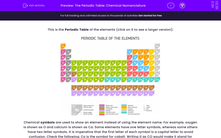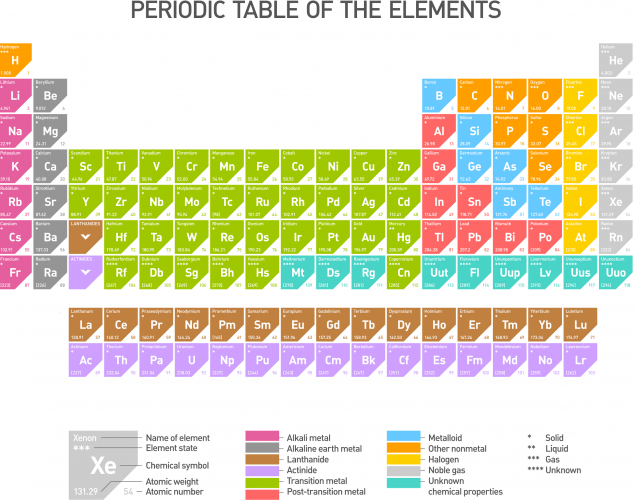# Name Chemical Compounds Using the Periodic Table

In this worksheet, students will learn how to name chemical compounds according to IUPAC (International Union of Pure and Applied Chemistry) rules.Key stage:  KS 3

Curriculum topic:   Chemistry: Chemical Reactions

Curriculum subtopic:   Formulae and Equations

Difficulty level:#### Worksheet Overview

This is the Periodic Table of the elements (click on it to see a larger version):Chemical symbols are used to show an element instead of using the element name. For example, oxygen is shown as O and calcium is shown as Ca.

Some elements have symbols of only one letter, whereas some others have two letter symbols. It is imperative that the first letter of each symbol is a capital letter to avoid confusion. Check the following: Co is the symbol for cobalt. Writing it as CO would make it stand for carbon monoxide, so there would be confusion in interpreting the symbol.

Chemical formulae

An atom is a stable chemical entity, a whole. An atom cannot be broken down by any chemical process. It is the smallest entity of an element that can be found in nature. Some elements can stand as atoms alone, whereas some others need to form molecules – two atoms together.

molecule refers to two or more atoms that have chemically bonded to make a stable entity. For example, sodium (Na) is found in nature as one atom.

Oxygen, on the other hand, cannot stand alone as one atom (O). The air we breathe in contains molecules of oxygen, i.e. two oxygen atoms bonded together. We refer to a molecule of oxygen as O2, where O shows that the element is oxygen and the subscript 2 shows we are referring to two oxygen atoms, which we have come to recognize as a molecule of oxygen. Note that it would be wrong to write O2. The number must always be at the bottom after the element that it refers to.Elements of the Periodic Table react and form compounds. We use chemical formulae to refer to those compounds. Formulae is the plural of the word 'formula'.

There are rules to follow when naming those compounds. The formula must show the atoms that the chemical compound contains. For example, carbon dioxide contains one carbon atom and two oxygen atoms. We write this as CO2. This clearly reflects the one carbon atom and the two oxygen atoms.Chemical formulae look more complicated for complex compounds. Potassium nitrate's formula is KNO3. Do not worry, but break it down slowly. K is the symbol for potassium (you may have to search the Periodic Table until you learn the main symbols). There is no number below it, so there is only one potassium atom in potassium nitrate. N is the symbol for nitrogen and no numbers below mean there is only one nitrogen atom. Finally, O is the symbol for oxygen. The number 3 is below it, so three oxygen atoms are found in potassium nitrate.

Compounds that are formed by two elements, a metal and a non-metal, are named by the name of the metal (the first symbol of the formula) followed by the name of the non-metal with the ending -ide. Let's look at some examples. NaCl is made of sodium (Na) and chlorine (Cl). Its name would be the name of the metal (sodium) followed by the name of the non-metal (chlorine) with -ide at the end - sodium chloride.

Have a go at naming the following compound, FeS, Fe is iron and S is sulfur.

Did you answer iron sulfide? Correct!

Let's have a go at some questions now.

If you need to look back at the introduction, just click on the red Help button.

### What is EdPlace?

We're your National Curriculum aligned online education content provider helping each child succeed in English, maths and science from year 1 to GCSE. With an EdPlace account you’ll be able to track and measure progress, helping each child achieve their best. We build confidence and attainment by personalising each child’s learning at a level that suits them.

Get started